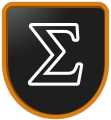# Math

VMK in this section are focused on analyzing and working through the mathematic formulae used to perform particular tasks. The main branches of mathematics used in these VMK are Algebra, Geometry, and Calculus. Each VMK will step you through the derivation and required calculations.

1: Lines in 2D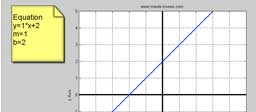In this video I explain how lines can be drawn in 2D and their general mathematical equation.
2: Parabolas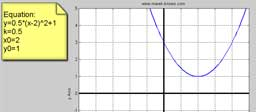In this video I explain the general equation used to define a parabola in 2D.
3: Perpendicular in 2D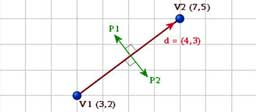This VMK shows you how to find a perpendicular line to a reference line. The reference line can be defined as two points or an equation.
4: Normals in 3D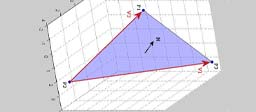You will be shown how to calculate the normal to a surface defined in 3D.
5: Change Range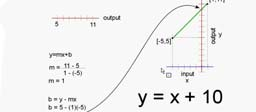The ability to map one set of numbers to another set of numbers is very useful in many applications. This VMK shows you how to set up a linear relationship between the input and the output values.
6: Unit Circle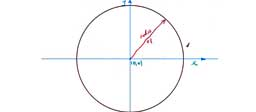In this VMK I show you how to calculate the (X,Y) coordinates for points located on a circle. I also briefly discuss the difference between degrees and radians.
7: Dot ProductIn this VMK we explore the dot product of a vector and how to use it to find the length of a vector, the angle between two vectors and the projection of one vector onto another.
8: Spherical Coordinate System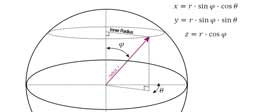The workings of the spherical coordinate system are explained and the three parameters: r, theta, phi are related to the standard cartesian x,y,z coordinates.
9: Rolling Ball using QuaternionsUsing the arrow keys, you can control a rolling ball on the screen. The quaternion equations used to make this happen is shown in this video. Visit the forum to download the finished application from this VMK.
10: Smooth Interpolation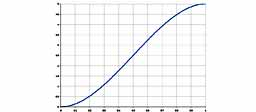In this video I show how to derive the equation used to create a smooth interpolation curve that connects two points. To see how to use this equation in C++ code, see Ghost Toast VMK 30.
X